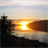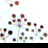# Select lines by length convert to polygon?

698
4
06-17-2014 06:17 AMby
New Contributor
You have to use python in the field calculator.  The basic operations are listed here under the Geometry Unit Conversion section.  The basic syntax for filling in length from lines is:

!shape.length!

You can convert units with expressions like:

!shape.length@yards!

Hi
I have a further question: I need calculate the mean of all this length fields and just want to transform lines that are less than mean value to be a polygon. How can I make this possible? I know there was a function called "features to polygon" but this is only work for whole line. Could you please kindly give me some tips? Thank you again!
Tags (3)
4 RepliesRegular Contributor II
To get the average line length interactively,
right click on the Shape_Length field in the attribute table
and click statistics.

let's say, for example, that the average is 5000 meters

then you create a feature layer of the lines feature class, with a where clause:
"Shape_Length" < 5000

You can make the layer interactively in ArcMap by adding that
statement above as a Query Definition to the layer,
or inside or outside of ArcMap by using the Make Feature layer tool
and setting the statement as the where clause or Expression

Now use the layer as the input to Feature To Polygon
Only the lines of less than the average length (in our example, 5000 meters)
will be used by the tool.by
New Contributor
To get the average line length interactively,
right click on the Shape_Length field in the attribute table
and click statistics.

let's say, for example, that the average is 5000 meters

then you create a feature layer of the lines feature class, with a where clause:
"Shape_Length" < 5000

You can make the layer interactively in ArcMap by adding that
statement above as a Query Definition to the layer,
or inside or outside of ArcMap by using the Make Feature layer tool
and setting the statement as the where clause or Expression

Now use the layer as the input to Feature To Polygon
Only the lines of less than the average length (in our example, 5000 meters)
will be used by the tool.

Hello, I have a further question. You said the mean value was calculated by clicking stastisic. however, in modelbuilder, summary stastastisic can only calculate mean value through output a new table file. How can I connect to this table file?

In my case, mean value is not a number rather it is a variable. for example, Shape_Length < table.mean (5000, 4000, 8000?). So.if it is possible to define mean value in table field? then this mean value can be used as a variable in Query definition?Regular Contributor II
Yes, instead of getting the statistics interactively, you can use the Summary Statistics tool
Yes, that tool produces an output table.
Yes, you have to look up the value of the MEAN for the field on which you requested statistics.
A search cursor will do that quickly (there will only be one record).
If you assign the value you have plucked from the table to a variable, you can use it in the subsequent where clause.
You never mentioned using model builder before, so I gave you the interactive workflow.
A Model Builder workflow will mirror the interactive one.by
New Contributor
Yes, instead of getting the statistics interactively, you can use the Summary Statistics tool
Yes, that tool produces an output table.
Yes, you have to look up the value of the MEAN for the field on which you requested statistics.
A search cursor will do that quickly (there will only be one record).
If you assign the value you have plucked from the table to a variable, you can use it in the subsequent where clause.
You never mentioned using model builder before, so I gave you the interactive workflow.
A Model Builder workflow will mirror the interactive one.

Hello.
Sorry for my miss mentioned. Could you please give me a simple example of how can that output mean value was defined or linked? if this is possible.
Thank you ❤️